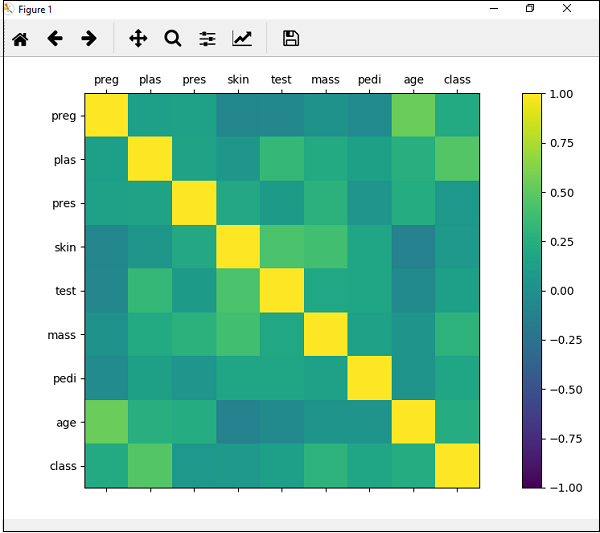# Machine Learning - Correlation Matrix Plot

Correlation is an indication about the changes between two variables. In our previous chapters, we have discussed Pearson’s Correlation coefficients and the importance of Correlation too. We can plot correlation matrix to show which variable is having a high or low correlation in respect to another variable.

## Example

In the following example, Python script will generate and plot correlation matrix for the Pima Indian Diabetes dataset. It can be generated with the help of corr() function on Pandas DataFrame and plotted with the help of pyplot.

from matplotlib import pyplot
import numpy
Path = r"C:\pima-indians-diabetes.csv"
names = ['preg', 'plas', 'pres', 'skin', 'test', 'mass', 'pedi', 'age', 'class']
data = read_csv(Path, names = names)
correlations = data.corr()
fig = pyplot.figure()
cax = ax.matshow(correlations, vmin=-1, vmax=1)
fig.colorbar(cax)
ticks = numpy.arange(0,9,1)
ax.set_xticks(ticks)
ax.set_yticks(ticks)
ax.set_xticklabels(names)
ax.set_yticklabels(names)
pyplot.show()


## OutputFrom the above output of correlation matrix, we can see that it is symmetrical i.e. the bottom left is same as the top right. It is also observed that each variable is positively correlated with each other.

machine_learning_with_python_understanding_data_with_visualization.htm